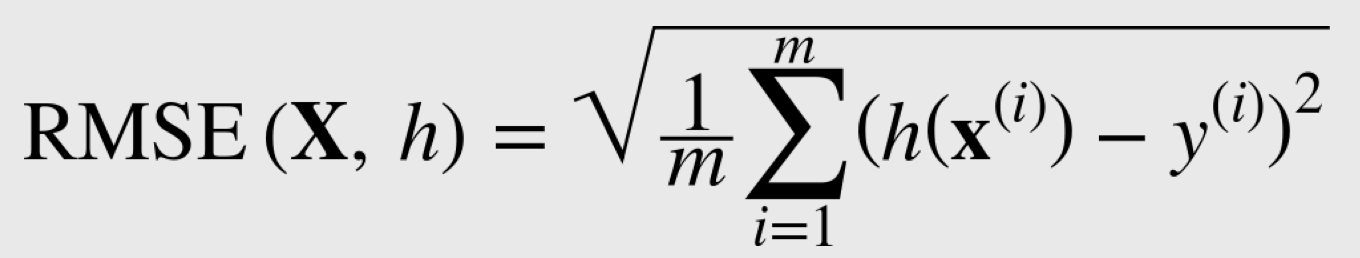# Attempting to Read Equations in Machine Learning

Nov 18, 2018

I ran into my first issue that caused me to stop and try to dissect what was happening. I’m not bad at math, but I’ve never studied calculus or something similar. I have taken algebra and trigonometry many years ago. I knew the math involved with machine learning would slow me down considerably. Especially since I am the type of person that likes to understand things on a deep level.

The equation was for Root Mean Square Error. I have been able to dissect it down to where I understand most of it. Here is what I know.

• m is the number of instances in the dataset. Here I am working with housing data.
• x(i) is a vector of all the feature values of the ith instance of the data set. So it works in conjunction with summation to iterate through each row of features in the data set.
• y(i) is the label for x(i).
• X is the matrix for all the feature values.
• h is a hypothesis function.So the equation says: Take each instance in a data set, run the features through the hypothesis function, subtract the labels, and square the result. Sum all the results and return. At least that’s what I think it says.

Just so know, what this equation does is determine how much error the prediction would have.

Here is what I don’t know. I don’t know what 1 over m is there for. I also don’t know if I need to understand this to proceed. I’m pretty sure scikit learn will do this for me. But it’s good to try to understand these things so I know what to use and when.